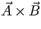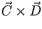# Problem: A) What is the magnitude of the cross product ?B) What is the direction of the cross product ?- into the page?- out of the page?- the magnitude is zero?C) What is the magnitude of the cross product ?D) What is the direction of the cross product ?- into the page?- out of the page?- the magnitude is zero?

###### FREE Expert Solution

Cross product:

$\overline{)\stackrel{\mathbf{⇀}}{\mathbf{A}}{\mathbf{×}}\stackrel{\mathbf{⇀}}{\mathbf{B}}{\mathbf{=}}\stackrel{\mathbf{⇀}}{\mathbf{A}}\stackrel{\mathbf{⇀}}{\mathbf{B}}{\mathbf{s}}{\mathbf{i}}{\mathbf{n}}{\mathbf{\theta }}}$

89% (278 ratings)###### Problem DetailsA) What is the magnitude of the cross product?

B) What is the direction of the cross product?

- into the page?

- out of the page?

- the magnitude is zero?

C) What is the magnitude of the cross product?

D) What is the direction of the cross product?

- into the page?

- out of the page?

- the magnitude is zero?